Couenne  0.2
Couenne::CouenneTwoImplied Class Reference

Cut Generator for implied bounds derived from pairs of linear (in)equalities. More...

#include <CouenneTwoImplied.hpp>

Inheritance diagram for Couenne::CouenneTwoImplied: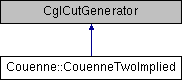## Public Member Functions

CouenneTwoImplied (CouenneProblem *, JnlstPtr, const Ipopt::SmartPtr< Ipopt::OptionsList >)
constructor More...

CouenneTwoImplied (const CouenneTwoImplied &)
copy constructor More...

~CouenneTwoImplied ()
destructor More...

CouenneTwoImpliedclone () const
clone method (necessary for the abstract CglCutGenerator class) More...

void generateCuts (const OsiSolverInterface &, OsiCuts &, const CglTreeInfo=CglTreeInfo()) const
the main CglCutGenerator More...

## Static Public Member Functions

static void registerOptions (Ipopt::SmartPtr< Bonmin::RegisteredOptions > roptions)

## Protected Attributes

CouenneProblemproblem_
pointer to problem data structure (used for post-BT) More...

JnlstPtr jnlst_
Journalist. More...

int nMaxTrials_
maximum number of trials in every call More...

double totalTime_
Total CPU time spent separating cuts. More...

double totalInitTime_
CPU time spent columning the row formulation. More...

bool firstCall_
first call indicator More...

int depthLevelling_
Depth of the BB tree where to start decreasing chance of running this. More...

int depthStopSeparate_
Depth of the BB tree where stop separation. More...

## Detailed Description

Cut Generator for implied bounds derived from pairs of linear (in)equalities.

Implied bounds usually work on a SINGLE inequality of the form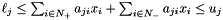where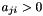for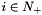and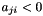for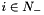, and allow one to infer better bounds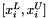on all variables with nonzero coefficients:

(1)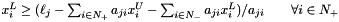(2)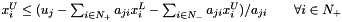(3)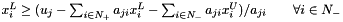(4)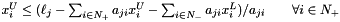Consider now two inequalities: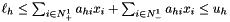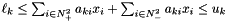and their CONVEX combination using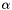and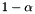, where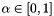: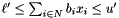with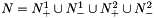,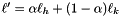, and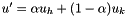. As an example where this might be useful, consider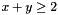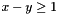with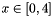and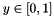. (This is similar to an example given in Tawarmalani and Sahinidis to explain FBBT != OBBT, I believe.) The sum of the two above inequalities gives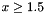, while using only the implied bounds on the single inequalities gives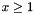.

The key consideration here is that the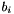coefficients,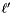, and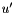are functions of, which determines which, among (1)-(4), to apply. In general,

if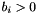then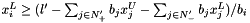,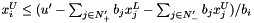;

if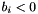then,.

Each lower/upper bound is therefore a piecewise rational function of, given thatand the content of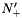and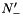depend on. These functions are continuous (easy to prove) but not differentiable at some points of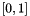.

The purpose of this procedure is to find the maximum of the lower bounding function and the minimum of the upper bounding function.

Divide the intervalinto at most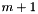intervals (where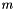is the number of coefficients not identically zero, or the number ofthat are nonzero for at least one value of). The limits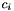of the subintervals are the zeros of each coefficient, i.e. the values ofsuch that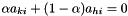, or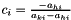.

Sorting these values gives us something to do on every interval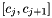when computing a new value of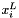and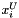, which I'll denote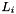and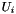in the following.

0) if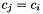then

• compute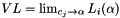• if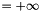, infeasible else compute derivative DL (should be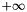)

1) else

• compute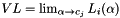(can be retrieved from previous interval as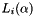is continuous)
• compute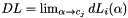update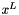with VL if necessary.

2) if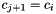then

• compute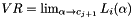• if =, infeasible else compute derivative DR (should be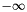)

3) else

• compute• compute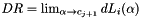updatewith VR if necessary.

if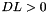and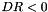, there might be a maximum in between, otherwise continue to next interval

compute internal maximum VI, updatewith VI if necessary.

Apply a similar procedure for the upper bound

This should be applied for any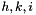, therefore we might have a lot to do. First, select possible pairs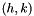among those for which there exists at least one variable that satisfies neither of the following conditions:

a) same sign coefficient, constraintsboth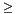or both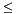b) opposite sign coefficient, constraints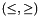or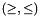as in those cases, nowould be inDefinition at line 174 of file CouenneTwoImplied.hpp.

## Constructor & Destructor Documentation

 Couenne::CouenneTwoImplied::CouenneTwoImplied ( CouenneProblem * , JnlstPtr , const Ipopt::SmartPtr< Ipopt::OptionsList > )

constructor

Referenced by clone().

 Couenne::CouenneTwoImplied::CouenneTwoImplied ( const CouenneTwoImplied & )

copy constructor

 Couenne::CouenneTwoImplied::~CouenneTwoImplied ( )

destructor

## Member Function Documentation

 CouenneTwoImplied* Couenne::CouenneTwoImplied::clone ( ) const
inline

clone method (necessary for the abstract CglCutGenerator class)

Definition at line 190 of file CouenneTwoImplied.hpp.

References CouenneTwoImplied().

 void Couenne::CouenneTwoImplied::generateCuts ( const OsiSolverInterface & , OsiCuts & , const CglTreeInfo = CglTreeInfo() ) const

the main CglCutGenerator

 static void Couenne::CouenneTwoImplied::registerOptions ( Ipopt::SmartPtr< Bonmin::RegisteredOptions > roptions )
static

## Member Data Documentation

 CouenneProblem* Couenne::CouenneTwoImplied::problem_
protected

pointer to problem data structure (used for post-BT)

Definition at line 208 of file CouenneTwoImplied.hpp.

 JnlstPtr Couenne::CouenneTwoImplied::jnlst_
protected

Journalist.

Definition at line 211 of file CouenneTwoImplied.hpp.

 int Couenne::CouenneTwoImplied::nMaxTrials_
protected

maximum number of trials in every call

Definition at line 214 of file CouenneTwoImplied.hpp.

 double Couenne::CouenneTwoImplied::totalTime_
mutableprotected

Total CPU time spent separating cuts.

Definition at line 217 of file CouenneTwoImplied.hpp.

 double Couenne::CouenneTwoImplied::totalInitTime_
mutableprotected

CPU time spent columning the row formulation.

Definition at line 220 of file CouenneTwoImplied.hpp.

 bool Couenne::CouenneTwoImplied::firstCall_
mutableprotected

first call indicator

Definition at line 223 of file CouenneTwoImplied.hpp.

 int Couenne::CouenneTwoImplied::depthLevelling_
protected

Depth of the BB tree where to start decreasing chance of running this.

Definition at line 226 of file CouenneTwoImplied.hpp.

 int Couenne::CouenneTwoImplied::depthStopSeparate_
protected

Depth of the BB tree where stop separation.

Definition at line 229 of file CouenneTwoImplied.hpp.

The documentation for this class was generated from the following file: